# KR100835759B1 - Image projector, inclination angle detection method, and projection image correction method - Google Patents

Image projector, inclination angle detection method, and projection image correction method Download PDF

## Info

Publication number
KR100835759B1
KR100835759B1 KR20067000923A KR20067000923A KR100835759B1 KR 100835759 B1 KR100835759 B1 KR 100835759B1 KR 20067000923 A KR20067000923 A KR 20067000923A KR 20067000923 A KR20067000923 A KR 20067000923A KR 100835759 B1 KR100835759 B1 KR 100835759B1
Authority
KR
South Korea
Prior art keywords
screen
measuring
angle
θ
surface
Prior art date
Application number
KR20067000923A
Other languages
Korean (ko)
Other versions
KR20060031685A (en
Inventor
히데아키 이노우에
Original Assignee
가시오게산키 가부시키가이샤
Priority date (The priority date is an assumption and is not a legal conclusion. Google has not performed a legal analysis and makes no representation as to the accuracy of the date listed.)
Filing date
Publication date
Priority to JPJP-P-2003-00275004 priority Critical
Priority to JP2003275004A priority patent/JP4155890B2/en
Application filed by 가시오게산키 가부시키가이샤 filed Critical 가시오게산키 가부시키가이샤
Publication of KR20060031685A publication Critical patent/KR20060031685A/en
Application granted granted Critical
Publication of KR100835759B1 publication Critical patent/KR100835759B1/en

• 238000003702 image correction Methods 0.000 title claims description 9
• 238000004364 calculation methods Methods 0.000 claims abstract description 28
• 238000004458 analytical methods Methods 0.000 claims description 23
• 230000000051 modifying Effects 0.000 claims description 11
• 238000009472 formulation Methods 0.000 claims 1
• 239000000203 mixtures Substances 0.000 claims 1
• 238000000034 methods Methods 0.000 description 2
• 238000006243 chemical reactions Methods 0.000 description 1

## Images

•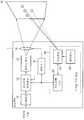•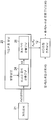•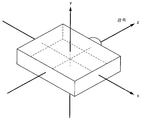•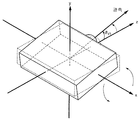•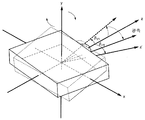•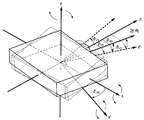••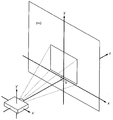•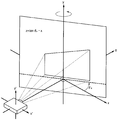•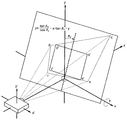•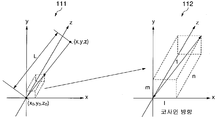••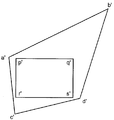•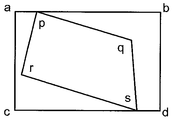•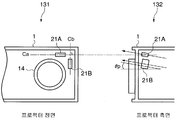••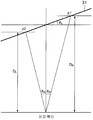## Classifications

• HELECTRICITY
• H04ELECTRIC COMMUNICATION TECHNIQUE
• H04NPICTORIAL COMMUNICATION, e.g. TELEVISION
• H04N9/00Details of colour television systems
• H04N9/12Picture reproducers
• H04N9/31Projection devices for colour picture display, e.g. using electronic spatial light modulators [ESLM]
• H04N9/3179Video signal processing therefor
• H04N9/3185Geometric adjustment, e.g. keystone or convergence
• GPHYSICS
• G03PHOTOGRAPHY; CINEMATOGRAPHY; ANALOGOUS TECHNIQUES USING WAVES OTHER THAN OPTICAL WAVES; ELECTROGRAPHY; HOLOGRAPHY
• G03BAPPARATUS OR ARRANGEMENTS FOR TAKING PHOTOGRAPHS OR FOR PROJECTING OR VIEWING THEM; APPARATUS OR ARRANGEMENTS EMPLOYING ANALOGOUS TECHNIQUES USING WAVES OTHER THAN OPTICAL WAVES; ACCESSORIES THEREFOR
• G03B21/00Projectors or projection-type viewers; Accessories therefor
• G03B21/14Details

## Abstract

In the projector to which the present invention is applied, the distance sensor 21 measures the distances between the projector 1 and the points on the plurality of screens 31. The angle calculation unit 23 obtains a formula representing the screen surface based on the plurality of distance data representing the measurement result by the distance sensor 21, and inclination angles θ H and θ of the screen 31 with respect to the optical axis of the projection light. V ) The angle calculation unit 23 supplies the obtained inclination angles θ H and θ V to the ladder correction unit 12. The ladder correction unit 12 performs ladder correction using the supplied inclination angles θ H and θ V.
Projector, screen, projected image, correction, tilt angle, distance sensor

## Description

IMAGE PROJECTOR, INCLINATION ANGLE DETECTION METHOD, AND PROJECTION IMAGE CORRECTION METHOD}

The present invention relates to a projector device, a tilt angle acquisition method of a projector, and a projection image correction method.

Projectors are devices for displaying images on the screen. The projector projects light towards the screen and displays the image on the screen.

If the projector is not tilted with respect to the ground and the screen is positioned perpendicular to the ground, the image is displayed without distortion on the screen.

However, if the projector is tilted with respect to the ground surface, the image on the screen is distorted. To prevent this, some conventional projectors have an acceleration sensor therein. The acceleration sensor detects the tilt angle of the projector with respect to the ground surface. The projector performs trapezoidal correction based on the detected tilt angle. Such a projector is disclosed, for example, in Japanese Unexamined Patent Application Publication No. 2001-339671 on page 3 and in FIG. 1.

In practice, however, the screen need not be perpendicular to the earth's surface.

Therefore, in order to determine the correction amount of the ladder correction, the inclination angle of the projector with respect to the ground surface and the inclination angle of the projector with respect to the axis perpendicular to the screen are required. If these inclination angles can be measured, ladder correction can be performed.

Conventional projectors thus correct the distorted appearance of the image to correct the appearance.

However, conventional projectors have the following problems.

First, as explained above, the inclination angles necessary for the ladder correction of the image are the inclination angles of the projector with respect to the ground surface, and the inclination angle of the projector with respect to the axis perpendicular to the screen. Therefore, even if the inclination angle of the projector with respect to the ground surface is detected, if the inclination angle of the projector with respect to the screen cannot be measured accurately or the measured result cannot be provided to the projector, ladder-type correction cannot be performed correctly.

Second, conventional projectors with acceleration sensors ignore the tilt angle of the screen relative to the projector in a direction parallel to the ground surface. In practice, there may be an inclination angle of the screen with respect to the projector in the horizontal direction. The acceleration sensor cannot detect the tilt angle of the screen with respect to the optical axis of the projected light. Therefore, the projectors with the conventional acceleration sensor cannot perform the ladder type correction based on the inclination angle of the screen with respect to the projector in the horizontal direction.

The detailed description of the documents set forth above is included herein.

SUMMARY OF THE INVENTION An object of the present invention is to provide a projector device capable of accurately correcting a projected image displayed on a screen, a method of obtaining a tilt angle of a projector, and a method of correcting image distortion.

One of the preferred embodiments of the present invention is a projector device, which measures a distance between a plurality of measuring points on the screen 31 and itself and outputs a plurality of distance data representing the measurement results. ;

Using a plurality of distance data output from the distance sensor 21, a screen formula indicating a positional relationship between the screen 31 on which the projection light is projected thereon and the optical axis of the projection light projected from the projector device 1 is obtained, An angle calculation unit 23 for obtaining an inclination angle of the optical axis with respect to the surface of the screen 31 using the obtained screen formula;

A correction unit 12 for correcting the distortion of the projected image displayed on the surface of the screen 31 using the inclination angle of the optical axis with respect to the surface of the screen 31 obtained by the angle calculating unit 23; And

And a light modulation unit 13 for converting the projection image corrected by the correction unit 12 into projection light and projecting the projection image onto the screen 31 surface.

Another one of the preferred embodiments of the present invention is a projector device, which measures the distance between each of a plurality of measuring points and each of them which is located above each of the plurality of measuring lines on the screen 31 that intersect each other, and measure A plurality of distance sensors 21A and 21B which respectively output a plurality of distance data representing the results;

Using the plurality of distance data output from the plurality of distance sensors 21A and 21B, respectively, the inclination angles of the plurality of measurement lines with respect to the surface perpendicular to the optical axis of the projection light projected from the projector device 1 are obtained, and the plurality of obtained An angle calculation unit 23 for obtaining an inclination angle of the optical axis with respect to the surface of the screen 31 by using the inclination angles of the optical element;

A correction unit 12 for correcting the distortion of the projection image to be displayed on the surface of the screen 31 by using the inclination angle of the optical axis with respect to the surface of the screen 31 obtained by the angle calculating unit 23; And

And a light modulation unit 13 for converting the projection image corrected by the correction unit 12 into projection light and projecting the projection image onto the screen 31 surface.

Another one of the preferred embodiments of the present invention is a method of obtaining an inclination angle for obtaining an inclination angle of the projector device 1 with respect to the surface of the screen 31,

A measuring step of measuring distances between the plurality of measuring points on the screen 31 and the projector device 1;

A screen which obtains a screen formula indicating the positional relationship between the surface of the screen 31 on which projection light is projected thereon and the optical axis of the projection light projected from the projector device 1 using a plurality of distance data representing the measurement result of the measurement step An official acquisition step; And

And an inclination angle obtaining step of obtaining an inclination angle of the surface of the screen 31 with respect to the optical axis by using the obtained screen formula.

Another one of the preferred embodiments of the present invention is a method of obtaining an inclination angle for obtaining an inclination angle of the projector device 1 with respect to the surface of the screen 31:

A measuring step of measuring distances between the plurality of measuring points and the projector device 1 positioned on each of the plurality of measuring lines on the screen 31 crossing each other;

An angle obtaining step of obtaining an angle formed between each of the plurality of measurement lines and a virtual screen surface perpendicular to the optical axis of the projector device 1; And

An inclination angle acquisition method comprising an analysis step of analyzing each angle obtained in the angle acquisition step as an inclination angle of the optical axis of the projector device 1 with respect to the surface of the screen 31.

Another one of the preferred embodiments of the present invention is a projection image correction method for correcting distortion of a projection image:

A measuring step of measuring distances between a predetermined reference point and a plurality of measuring points on the screen 31;

A screen formula acquiring step of obtaining a screen formula indicating a positional relationship between the optical axis of the projection light projected onto the screen 31 and the screen 31 using a plurality of distance data representing the measurement result of the measuring step;

An inclination angle obtaining step of obtaining an inclination angle of the optical axis with respect to the surface of the screen 31 using the obtained screen formula; And

And a correction step of correcting the distortion of the projection image to be displayed on the screen 31 using the inclination angle of the optical axis with respect to the surface of the screen 31 obtained in the inclination angle obtaining step.

Another one of the preferred embodiments of the present invention is a projection image correction method for correcting distortion of a projection image:

A measuring step of measuring distances between the plurality of measuring points and the projector device 1 positioned on each of the plurality of measuring lines on the screen 31 crossing each other;

An angle obtaining step of obtaining an angle formed between each of the plurality of measurement lines and a virtual screen surface perpendicular to the optical axis of the projector device 1;

An angle analysis step of analyzing each angle obtained in the angle obtaining step as an inclination angle of the optical axis of the projector device 1 with respect to the surface of the screen 31; And

Projection image correction comprising a correction step of correcting the distortion of the projection image to be displayed on the screen 31 surface by using the inclination angles of the optical axis of the projector 1 with respect to the surface of the screen 31 obtained by the analysis in the angle analysis step. It is a way.

The projector to which the present invention is applied can accurately perform correction of a projected image whose focus is matched on a screen.

These and other objects and advantages of the present invention will become more apparent from the following detailed description and understanding of the accompanying drawings, in which:

1 is a block diagram showing the structure of a projector according to a first embodiment of the present invention;

FIG. 2 is a block diagram showing the structure of the angle calculating unit shown in FIG. 1;

3 is a diagram illustrating a coordinate system of a projector;

4 is a view showing a state in which the projector rotates around the x-axis of the coordinate system of FIG. 3;

FIG. 5 is a diagram illustrating a state in which the projector rotates around the y axis after the projector rotates around the x axis of the coordinate system of FIG. 3;

6 is a diagram for explaining a method for obtaining an inclination angle of a screen with respect to an optical axis;

FIG. 7 is a diagram for explaining the positional relationship between the image on the screen and the inclination angle shown in FIG. 6;

8 is a diagram showing a coordinate system set on a screen;

FIG. 9 is a view showing a state in which the screen is rotated around the y axis of the coordinate system shown in FIG. 8; FIG.

FIG. 10 is a diagram showing a state in which the screen rotates around the y axis after the screen is rotated around the y axis of the coordinate system shown in FIG. 8;

11 is a diagram for explaining a method of obtaining coordinates of a measured point based on distance data;

12A to 12C are views showing the operation of the ladder correction unit and the light modulation unit shown in FIG. 1;

Fig. 13 is a diagram showing the arrangement of distance sensors included in the projector according to the second embodiment of the present invention, in particular showing the front side (left side view) of the projector and the side side (right side view) of the projector;

14 is a block diagram showing the structure of an angle calculating unit constituting the projector according to the second embodiment;

FIG. 15 is a view for explaining a calculation method performed by the angle calculating unit shown in FIG. 14 for calculating an angle based on two distance data indicating a distance measured by one distance sensor.

Projectors in accordance with embodiments of the present invention will be described with reference to the drawings.

(First embodiment)

The projector 1 according to the first embodiment includes a scaler 11, a ladder correction unit 12, an optical modulation unit 13, a projection lens 14, an optical instrument 15, and a screen angle sensor (16) is provided.

The scaler 11 adjusts the resolution of the image signal.

The ladder correction unit 12 performs ladder correction on an image signal having a resolution corrected by the scaler 11.

The ladder correction unit 12 projects the projection after correction in the projection image before correction projected on the screen 31 based on the inclination angles θ V and θ H of the surface of the screen 31 supplied from the screen angle sensor 16. Set the position and shape of the image. Subsequently, the ladder correction unit 12 temporarily deforms the image signal continuously and performs the ladder deformation. The inclination angle θ V indicates the inclination of the screen 31 in the direction perpendicular to the optical axis of the projection light. The inclination angle θ H indicates the inclination angle of the screen 31 in the horizontal direction with respect to the optical axis.

The ladder correction unit 12 obtains a correction amount for correcting the projection image according to an analysis table for interpreting the inclination angles θ V and θ H as a correction amount for correcting the projection image. Subsequently, the ladder correction unit 12 outputs the image signal after the correction. When using an analysis table, the ladder correction unit 12 has a memory for storing a pre-generated analysis table. Instead, the ladder correction unit 12 may be configured to perform ladder correction using a formula representing the screen plane whenever the inclination angles θ V and θ H are supplied. In order to generate the analysis table, (1) the square shape before correction is obtained based on the inclination angles θ V and θ H and the magnification; (2) the rectangle obtained after correction is defined in the rectangle before correction, and the coordinates of the rectangle obtained after correction are obtained; (3) the coordinates of the square before correction are obtained based on the coordinates of the square obtained after correction by the inverse transformation of the coordinates, whereby the conversion scheme is determined; (4) A circuit parameter is derived from the coordinates obtained by the inverse transformation (this parameter is derived in advance and stored in memory as an analysis table). If no interpretation table is used, the same correction operation is realized by performing the processes of (1) to (4) as necessary.

The light modulation unit 13 converts the corrected image signal output from the ladder correction unit 12 into projection light. For example, a transmissive or reflective liquid crystal panel or a digital micromirror device (DMD) is used as the light modulation unit 13.

The projection lens 14 displays on the screen 31 an image which has been converted into light by the light modulation unit 13 from the image signal after correction.

The optical mechanism 15 adjusts the focus and the like of the projection lens 14 based on the inclination angles θ V and θ H detected by the screen angle sensor 16. When the projector 1 is provided with an enlargement mechanism, the optical mechanism 15 also performs magnification control and provides the ladder type correction unit 12 with information about the magnification. The magnification of the projector 1 is a value obtained by multiplying the ratio of the projection distance and the size of the projection image by a constant (the magnification is proportional to the width / projection distance of the projection image = 2 x tan (half the viewing angle)).

The magnification variable range in which the magnification can be changed is wide, and the change of the ladder-type correction correction amount based on the magnification is not negligible. Therefore, the ladder correction unit 12 performs ladder correction using an enlarged magnification supplied from the optical mechanism 15.

However, when the projector 1 does not have an enlargement mechanism or when the projector 1 has an enlargement mechanism but the enlarged variable range is narrow, the ladder correction unit 12 regards the enlarged magnification as a constant and corrects the ladder type correction. Do this.

The screen angle sensor 16 detects the inclination angles θ V and θ H of the surface of the screen 31 with respect to the optical axis of the light projected by the projector 1. The screen angle sensor 16 includes a distance sensor 21, a control unit 22, and an angle calculation unit 23.

The distance sensor 21 measures the distances between the projector 1 and the plurality of measuring points on the screen 31. The distance sensor 21 outputs distance data indicating the measurement results. It is sufficient if the distance sensor 21 can measure the distances between the projector 1 and at least three measuring points on the screen 31. The distance sensor 21 may be active or passive.

The control unit 22 controls to measure the distance between the projector 1 and the points on the plurality of screens 31. To measure the distance, the control unit 22 projects an infrared light spot (in the case of active type) or a predetermined image pattern (passive type) on the screen 31.

The angle calculating unit 23 displays the inclination angle on the screen 31 based on the distance data indicating the distance between the projector 1 measured by the distance sensor 21 and the plurality of measurement points on the screen 31. θ V and θ H ) are calculated. The angle calculating unit 23 is configured of, for example, a digital signal processor (DSP) or a computer. As shown in FIG. 2, the angle calculating unit 23 includes a screen formula calculating unit 24 and an inclination angle calculating unit 25.

The screen formula calculating unit 24 derives a formula for displaying the screen plane of the screen 31 based on the distance data for the plurality of measuring points. The inclination angle calculating unit 25 calculates the inclination angles θ V and θ H of the screen 31 by using a formula representing the screen plane derived through the screen formula calculating unit 24.

Here, the inclination angles θ V and θ H will be described in each case when the origin of the coordinate system is set at the center of the projector 1 and when the origin of the coordinate system is set at the surface of the screen 31. The coordinate system is a three-dimensional rectangular coordinate system composed of x, y, and z axes.

First, the case where the origin of the coordinate system is set at the center of the projector 1 as shown in FIG. 3 will be described. If screen 31 is perpendicular to the z-axis and parallel to the x-axis, y-axis, the screen is at a position z = d. 3 to 7 are shown so that the position of the incident pupil of the projection lens 14 coincides with the origin of the coordinate system.

Consider the case where the screen 31 is not tilted and the projector 1 is tilted. In this case, it is assumed that the screen 31 exists at the position where z = d.

At this time, attention should be paid to the tilting order of the projector 1. When the projector 1 is inclined, first, the projector 1 is inclined by the inclination angle θ V1 around the x-axis as the rotation axis. Subsequently, the projector 1 is inclined by the inclination angle θ H1 around the y axis as the rotation axis. The z 'axis of FIG. 5 is reached by tilting the z axis by the inclination angle θ H1 around the y axis as the rotation axis. The optical axis of the projection light from the projector 1 is above the z 'axis in the range of z> 0.

The inclination angle θ V1 shown in FIGS. 4 and 5 is an angle formed between the optical axis and the z axis of the light projected from the projector 1 when the projector 1 is rotated around the x axis. The inclination angle θ H1 shown in FIG. 5 is an angle formed between the z axis and the z ′ axis mapped to the zx plane of the optical axis of the light projected from the projector 1 when the projector 1 is further rotated around the y axis. .

When the rotation order is reversed, i.e. as shown in Figs. 6 and 7, the projector 1 is inclined by the inclination angle θ H2 around the y axis as the rotation axis and then the inclination angle θ V2 around the x 'axis as the rotation axis. When inclined by, the values of the inclination angles θ H1 and θ H2 are equal to the values of the inclination angles θ V1 and θ V2 , respectively.

However, when the projector 1 is first rotated around the y axis as the rotation axis, the rotation axis where the projector 1 is next rotated around it is reached by tilting the x axis around the y axis as the rotation axis by the inclination angle θ H2 . 'wet.

6 and 7, even when the rotation order is reversed, it is known that the same state appears when the rotation axis is not wrong.

In FIG. 7, "d" represents the distance between the center of the projector 1 and the screen 31. The two rectangles on the screen 31 are projected images after the projector 1 is rotated. The rectangle a'b'c'd 'represents a projection image when the ladder correction unit 12 does not perform ladder correction. The rectangle p'q'r's' represents the projection image after the ladder correction unit 12 performs the ladder correction.

As shown in FIG. 7, the rectangle a'b'c'd 'is converted into a rectangle p'q'r's' by the ladder correction unit 12 performing the ladder correction.

The point "t" represents the temporary intersection of the optical axis of the projector 1 and the screen 31 when the projector 1 is first rotated around the y axis by the inclination angle θ H2 . The point “u” denotes the optical axis and screen 31 of the projector 1 when the projector 1 first rotates around the x axis by the inclination angle θ V1 and then rotates around the y axis by the inclination angle θ H1 . ) Represents the actual intersection of. The point "v" represents the intersection of the two diagonals of the rectangle a'b'c'd '.

As shown in FIG. 7, when the point "u" is located directly above the point "t" which is a temporary intersection, the point "v" is located directly above the point "u". Therefore, the description will be easier to understand if the inclination angles are represented by (θ V2 and θ H2 ).

Next, the case where the screen 31 is inclined instead of the projector 1 will be described.

8 shows the state before the screen 31 is tilted. In order to facilitate understanding, the coordinate system is set as shown in FIG. In particular, the origin of the coordinate system is set to the point O on the screen 31. For ease of understanding, the point O is assumed to be the intersection of the optical axis of the light projected from the projector 1 and the screen 31.

In this case, the formula representing the screen plane is Z = 0.

As the screen 31 is rotated around the y axis by the inclination angle θ H , as shown in FIG. 9, the plane of the screen 31 is represented by the following equation (15).

When the screen 31 is further rotated around the x-axis by the inclination angle θ V as shown in FIG. 10, equation (15) is changed to the following equation (16).

The problem is the rotation order. It is necessary to reverse the rotation order of the projector 1 as shown in Figs. That is, as shown in FIGS. 9 and 10, the screen 31 is first rotated around the y axis by the inclination angle θ H and then rotated around the x axis by the inclination angle θ V.

Thus, the inclination angles θ H and θ V of equation (16) coincide with the inclination angles θ H1 and θ V1 shown in FIGS. 6 and 7. That is, in the case where the origin of the coordinate system is set at the center of the projector 1 and the projector 1 is inclined, and in the case where both the origin of the coordinate system is set on the surface of the screen 31 and the screen 31 is inclined, the projector The inclination angles θ H1 and θ V1 in (1) and the inclination angles θ H and θ V of the screen 31 coincide with each other.

Next, a method of calculating the inclination angles θ H and θ V based on the distance data representing the distance between the projector 1 and the measurement point on the screen 31 is described.

The plane of the screen 31 is represented by the following equation (17). The coordinate system used here is a three-dimensional rectangular coordinate system whose origin is the center of the projector 1 and consists of x, y, and z axes.

(a, b, c are constants)

If there is direction information for the preset direction, a plurality of distance data, three-dimensional coordinates (x, y, z) for the measuring point on the screen 31 can be obtained. If there are three sets of direction information and distance data, three sets of three-dimensional coordinates {(x1, y1, z1), (x2, y2, z2), (x3, y3, z3)} can be obtained.

In this case, cubic system equations are constructed based on equation (17). The coefficients a b and c of equation (17) can be obtained by solving these simultaneous equations. It is not necessary to find "c" because the coefficient "c" represents the parallel movement of the z axis.

By comparing equations (16) and (17), the coefficients "a" and "b" are represented by the following equation (18).

According to equation (18), the inclination angles θ V and θ H are represented by the following equation (19).

Using equation (19) the inclination angles θ H and θ V can be obtained.

Next, a specific method of obtaining the inclination angles θ H and θ V based on the plurality of distance data is described.

In order to obtain the inclination angles θ H and θ V based on the plurality of distance data, (1) the coordinates of the measuring point are obtained using the distance data, and (2) the screen based on the coordinates of the measuring points. An equation representing the plane is obtained, and (3) inclination angles θ H and θ V are obtained based on the equation representing the screen plane. These processes are described in order.

(1) the acquisition of the coordinates of the measuring points using distance data

Coordinates of already set light projection points of the distance sensor 21 are indicated by (x0, y0, z0), as indicated by " 111 " in FIG. 11, and direction cosine in the direction in which the distance sensor 21 projects light. Denotes "l", "m", and "n" as indicated by "112" in FIG. In this case, the coordinates (x, y, z) of the measuring point are represented by the following equation (20). "L" in FIG. 11 represents the distance between the projector 1 and the measurement point obtained based on the distance data. Light projection point means the light emission point of the active distance sensor.

x = x0 + L × l,

y = y0 + L × m,

z = z0 + L × n

(2) Using the coordinates of the measured points, we obtain an equation representing the screen plane of the screen 31 onto which light is projected.

If there are three measurement points, the three-dimensional coordinates of the three points are (x1, y1, z1), (x2, y2, z2), (x3, y3, z3), respectively. In this case, equation (21) is formed according to equation (17) which is the following simultaneous equations representing the screen plane.

Solving this system of equations 21, the coefficients a, b, c are represented by the following equation (22).

If there are three or more measurement points, the coarse resolution of the screen plane can be obtained by means of the least-square method.

In this case, if the coordinates of the measuring points are (xi, yi, zi), s0, ..., s8 are obtained by equation (23).

If there are three or more measurement points, the screen plane is represented by the following equation (24) using s0, ..., s8 obtained from equation (23).

Solving equation (24) with respect to coefficients (a, b, c), these coefficients are represented by the following equation (25).

If there are three measuring points, solving equation (24) is equivalent to solving the system of equations (21).

(3) Obtain the inclination angles θ H and θ V

Tilt angles θ H and θ V are obtained through equation (19) above.

As described above, the inclination angles θ H and θ V can be obtained based on a plurality of distance data using equations (19-25).

The screen formula calculating unit 24 calculates coefficients "a" and "b" according to equations (20 to 25), and calculates the calculated coefficients "a" and "b" to the inclination angle calculating unit ( 25).

The inclination angle calculation unit 25 calculates the inclination angles θ H and θ V using the coefficients “a” and “b” supplied according to equation (19). The angle calculation unit 23 supplies the inclination angles θ H and θ V calculated by the inclination angle calculation unit 25 to the ladder correction unit 12.

The angle calculation unit 23 may be configured to calculate the inclination angles θ H and θ V in advance according to equations (19 to 25). In this case, the inclination angle calculation unit 25 has a memory and stores in the memory a pre-generated analysis table according to equations (19 to 25) for coupling the plurality of distance data to the inclination angles θ H and θ V. do.

Next, the operation of the projector 1 according to the first embodiment is explained.

Under the control of the control unit 22, the distance sensor 21 of the screen angle sensor 16 measures the distance between the projector 1 and a plurality of points on the screen 31. The distance sensor 21 supplies the distance data indicating the distance measurement result to the angle calculation unit 23.

As described above, the equation calculating unit 24 of the screen of the angle calculating unit 23 calculates coefficients a and b according to the equations 20 to 25. The inclination angle calculation unit 25 calculates the inclination angles θ H and θ V of the screen 31 using the equation (19) and the calculated coefficients (a, b).

The angle calculation unit 23 supplies the inclination angles θ V and θ H calculated by the inclination angle calculation unit 25 to the ladder correction unit 12.

When the inclination angles θ V and θ H are supplied to the ladder correction unit 12, the scaler 11 adjusts the resolution of the image signal and supplies the adjusted image signal to the ladder correction unit 12.

The image is assumed to be an abcd shown in FIG. 12A. In this case, the ladder correction unit 12 performs ladder correction on this rectangle abcd based on the inclination angles θ H and θ V supplied from the screen angle sensor 16.

Ladder-correction unit 12 is interpreted to combine the computed using representing the supplied inclination angle (θ H and θ V) and the plane of the screen expression inclination angle (θ H and θ V) and the information amount for the projected image By using the table, a correction amount for the projected image is obtained.

However, as described above, the ladder correction unit 12 may perform ladder correction each time the inclination angles θ H and θ V are supplied without using an analysis table.

The ladder correction unit 12 determines the position and shape of the projection image obtained as a result of correction based on the correction amount obtained using the image projected on the screen 31. Subsequently, the ladder correction unit 12 performs ladder correction by temporarily projecting and deforming the image signal.

As a result of the ladder correction performed by the ladder correction unit 12, the rectangle abcd shown in Figs. 12A and 12C is corrected to the rectangle pqrs shown in Fig. 12C.

The light modulation unit 13 is obtained after correction by the ladder-type correction unit 12 and converts an image signal representing a square pqrs in FIG. 12C into projection light. The projection light obtained by switching by the optical modulation unit 13 is projected onto the inclined screen 31 by the inclination angles θ H and θ V. Thus, the projected image after the ladder correction, that is, the rectangle p'q'r's' shown in FIG. 12B, is projected onto the screen 31. The square a'b'c'd 'before correction shown in FIG. 12B is a projection image when the ladder correction unit 12 does not perform ladder correction.

When the projector 1 has a magnification mechanism, the optical mechanism 15 performs magnification control and supplies information on the magnification to the ladder correction unit 12. In this case, the ladder correction unit 12 performs ladder correction based on the inclination angles θ H and θ V and the magnification.

When the projector 1 projects the rectangle pqrs shown in Fig. 12C on the screen 31 through the projection lens 14, the rectangle p'q'r's' shown in Fig. 12B is placed on the screen 31. Is displayed.

As described above, according to the first embodiment, the distances between the projector 1 and the plurality of measuring points on the screen 31 are measured using the distance sensor 21. The equation representing the screen plane is then derived based on the measured distances and the tilt angles θ H and θ V are obtained.

Thus, the inclination angles θ H and θ V can be obtained without the use of an acceleration sensor. That is, the ladder correction can be performed without calculating the inclination angle of the screen with respect to the ground surface. Further, ladder correction can be accurately performed when the screen 31 is inclined in the horizontal direction with respect to the optical axis and when the screen 31 is inclined in the horizontal and vertical directions.

(Second embodiment)

The projector according to the second embodiment includes two distance sensors for measuring the distance between the projector and the plurality of measuring points arranged in the horizontal direction on the screen and the distance between the projector and the plurality of measuring points arranged in the vertical direction on the screen. And tilt angles are obtained using these sensors.

The structure of the projector 1 according to the second embodiment is shown in FIG.

The projector 1 according to the second embodiment has distance sensors 21A and a distance sensor 21B as distance sensors, which are indicated by (131, 132) in FIG.

The distance sensors 21A and 21B are both phase difference sensors having multiple measurement functions capable of measuring distance in a plurality of directions, for example, configured as an AF module.

The distance sensors 21A and 21B are disposed near the projection lens such that each center line Ca and Cb vertically intersects, as indicated by reference numeral 131 in FIG. 13. The distance sensors 21A and 21B measure the distances between the projector 1 and a plurality of measurement points arranged on measurement lines on the screen corresponding to the respective center lines Ca and Cb.

As indicated by reference numeral 132 in FIG. 13, the rising angles of the distance sensors 21A and 21B with respect to the optical axis of the projection light of the projector 1 are assumed to be θ P. The elevation angle θ P has a positive value or a negative value and may be zero.

As shown in FIG. 14, the angle calculation unit 23 according to the second embodiment includes an inclination angle calculation unit 25 and an angle analysis unit 26.

The inclination angle calculation unit 25 according to the second embodiment calculates the inclination angles θ A and θ B based on the distance representing the measurement result performed by the distance sensors 21A and 21B. The inclination angles θ A and θ B are the inclination angles of the screen 31 with respect to the center lines Ca and Cb, respectively.

If the distances between the projector 1 and the plurality of measuring points arranged on the measuring lines corresponding to the center lines Ca and Cb can be measured, the inclination angles in the horizontal and vertical directions of the screen 31 can be obtained. .

The principle of obtaining the tilt angles is now described with reference to FIG. 15.

The inclination angle θ S of the screen 31 with respect to the optical axis of the projection light emitted from the projector 1 is represented by the following equation (26).

(D R is the distance between the projector 1 and the measuring point P1 on the measuring line, D L is the distance between the projector 1 and the measuring point P2 on the measuring line, θ W is from the projector 1 Is an angle formed between the optical axis of the projection light and the connecting line of the measuring point P1 and the projector 1, and is an angle formed between the line connecting the projector 1 and the measuring point P2 and the optical axis)

It is assumed that the inclination angle θ S obtained from the measurement result of the distance sensor 21A is θ A , and the inclination angle θ S obtained from the measurement result of the distance sensor 21b is θ B. Since the distance sensors 21A and 21B are disposed such that the center lines Ca and Cb cross vertically, the measurement lines of the distance sensors 21A and 21B cross vertically. Therefore, the inclination angles θ V and θ H can be calculated based on the following equation (27) based on the inclination angles θ A and θ B.

The inclination angle calculation unit 25 calculates the inclination angles θ A and θ B in accordance with equation (26), and the angle analysis unit 26 calculates the inclination angles θ V and θ H in accordance with equation (27). do.

The angle analysis unit 26 interprets the inclination angles θ A and θ B calculated by the inclination angle calculation unit 25 as the inclination angles θ V and θ H , respectively, according to equation (27). Thus, the angle analysis unit 26 obtains the inclination angles θ V and θ H.

The angle calculation unit 23 supplies the inclination angles θ V and θ H calculated by the angle analysis unit 26 to the ladder correction unit 12.

Next, the operation of the projector 1 according to the second embodiment will be described.

The control unit 22 controls the distance sensor 21A for measuring the distances between the projector 1 and a plurality of measuring points arranged in the horizontal direction.

In addition, the control unit 22 controls the distance sensor 21B for measuring the distances between the projector 1 and the plurality of measuring points arranged in the vertical direction.

The distance sensors 21A and 21B supply distance data indicating the measured distance between the projector 1 and the respective measuring points to the angle calculation unit 23.

The inclination angle calculation unit 25 of the angle calculation unit 23 calculates the inclination angles θ A and θ B using equation (26) based on the distance data supplied from the distance sensors 21A and 21B.

The angle analysis unit 26 interprets the inclination angles θ A and θ B as the inclination angles θ V and θ H in accordance with equation (27).

The angle calculation unit 23 supplies the inclination angles θ V and θ H calculated by the angle analysis unit 26 to the ladder correction unit 12.

The ladder correction unit 12 corrects the distortion of the projected image based on the inclination angles θ V and θ H supplied from the angle calculation unit 23.

As described above, according to the second embodiment, the two distance sensors 21A, 21B determine the distances between the projector 1 and the plurality of measurement points arranged on measurement lines perpendicularly intersecting on the screen 31. Measure The angle calculation unit 23 calculates the inclination angles θ V and θ H based on the plurality of distance data.

Therefore, the inclination angles θ V and θ H in the horizontal and vertical directions can be calculated by providing two distance sensors 21A and 21B capable of measuring distances in a plurality of directions, respectively, and the number of sensors You can reduce the projector by reducing the In addition, the projector 1 can be manufactured at low cost. In addition, by using the distance sensors 21A and 21B, the distance measurement pattern can be projected through the projection lenses 14, and the measurement accuracy can be improved.

Various embodiments may be considered in order to implement the present invention in addition to the first and second embodiments described above.

For example, in the first and second embodiments described above, the projector 1 may include an acceleration sensor in addition to the distance sensors 21A and 21B. If the distance cannot be accurately measured by the distance sensors 21A and 21B, the projector 1 may be configured to perform ladder correction using the acceleration sensor based on the inclination angle θ V in the vertical direction.

In addition, in the above-described embodiments, the explanation has been made using a three-dimensional rectangular coordinate system set at the center of the projector 1 or set on the plane of the screen 31. However, as long as the projector 1 and the screen 31 exist in the space defined by the three-dimensional rectangular coordinate system, the inclination angles θ V and θ H can be obtained regardless of the position at which the origin is set. In addition, the inclination angles θ V and θ H can be similarly obtained without using a three-dimensional rectangular coordinate system, for example, using a polar coordinate system.

Various embodiments and changes may be made to the present invention without departing from the broad spirit and scope of the invention. The above described embodiments are intended to illustrate the present invention and are not intended to limit the scope of the present invention. The scope of the invention is represented by the appended claims rather by the embodiments. Various modifications made within the meaning of the equivalents of the claims of the invention and within the claims are considered to be within the scope of the invention.

Thus, according to the present invention, the projection image displayed on the screen can be corrected accurately.

## Claims (10)

1. delete
2. A distance sensor 21 that measures distances between the plurality of measuring points on the screen 31 and itself and outputs a plurality of distance data representing the measurement results;
Using a plurality of distance data output from the distance sensor 21, a screen formula indicating a positional relationship between the screen 31 on which the projection light is projected thereon and the optical axis of the projection light projected from the projector device 1 is obtained. An angle calculating unit 23 for obtaining an inclination angle of the optical axis with respect to the surface of the screen 31 using the obtained screen formula;
A correction unit (12) for correcting the distortion of the projected image displayed on the surface of the screen (31) using the inclination angle of the optical axis with respect to the surface of the screen (31) obtained by the angle calculating unit (23);
A light modulation unit (13) for converting the projection image corrected by the correction unit (12) into projection light and projecting the projection image onto the surface of the screen (31); And
An optical mechanism 15 for controlling magnification and providing information on the magnification to the correction unit 12;
The correction unit 12 corrects the distortion of the projection image to be displayed on the surface of the screen 31 by using the information about the magnification supplied from the optical device 15 and the inclination angle of the optical axis with respect to the surface of the screen 31. And a projector configured to correct.
3. A plurality of distance sensors for measuring the distance between each of the plurality of measuring points and the respective itself positioned on each of the plurality of measuring lines on the screen 31 intersecting each other, and outputs a plurality of distance data representing the measurement results, respectively (21A, 21B);
Acquiring and obtaining the inclination angles of the plurality of measurement lines with respect to the surface perpendicular to the optical axis of the projection light projected from the projector device 1 by using the plurality of distance data respectively output from the plurality of distance sensors 21A and 21B. An angle calculation unit 23 for obtaining an inclination angle of the optical axis with respect to the surface of the screen 31 using the plurality of tilt angles;
A correction unit (12) for correcting the distortion of the projection image to be displayed on the surface of the screen (31) by using the inclination angle of the optical axis with respect to the surface of the screen (31) obtained by the angle calculating unit (23); And
And a light modulation unit (13) for converting the projection image corrected by the correction unit (12) into projection light and projecting the projection image onto the surface of the screen (31).
4. The method of claim 3, wherein
It further comprises an optical instrument 15 for controlling the magnification and providing information on the magnification to the correction unit 12,
The correction unit 12 corrects the distortion of the projection image to be displayed on the surface of the screen 31 by using the information about the magnification provided from the optical device 15 and the inclination angle of the optical axis with respect to the surface of the screen 31. And a projector configured to correct.
5. As an inclination angle acquisition method for acquiring an inclination angle of the projector device 1 with respect to the surface of the screen 31:
A measuring step of measuring distances between the plurality of measuring points on the screen (31) and the projector device (1);
A screen formula indicating the positional relationship between the surface of the screen 31 on which projection light is projected thereon and the optical axis of the projection light projected from the projector device 1 using a plurality of distance data representing the measurement result of the measurement step Obtaining a screen formula; And
And an inclination angle obtaining step of obtaining an inclination angle of the surface of the screen (31) with respect to an optical axis by using the obtained screen formula.
6. 6. The screen formulation obtaining step according to claim 5, wherein the screen formula acquiring step is composed of x, y, z axes perpendicular to each other in the space where the projector device 1 and the screen 31 exist and the screen 31 is setting a three-dimensional rectangular coordinate system perpendicular to the z-axis, expressing the screen formula by equation (1) including a, b, and c as coefficients, measuring a plurality of measurement points obtained in the measuring step Assigning the coordinates (xi, yi, zi) to x, y, z of Equation (1), using the system of equations (2) obtained by the assignment step, according to equation (3). obtaining s8), obtaining coefficients (a, b, c) of equation (1) according to equation (4), and assigning the obtained coefficients (a, b, c) to equation (1) Obtaining the screen formula represented by equation (1); And
In the obtaining of the inclination angle, the screen formula and the screen 31 obtained in the screen formula obtaining step are obtained when the screen is rotated by the inclination angle θ H about the y axis and by the inclination angle θ V about the x axis. Obtaining angles of inclination (θ H and θ V ) using equation (5) representing the point coordinates (x, y, z) above.
[Equation 1]

[Equation 3]
[Equation 4]
[Equation 5]
7. As an inclination angle acquisition method for acquiring the inclination angle of the projector device 1 with respect to the surface of the screen 31:
A measuring step of measuring distances between the plurality of measuring points located on each of the plurality of measuring lines on the screen (31) crossing each other and the projector device (1);
An angle obtaining step of obtaining an angle formed between each of the plurality of measurement lines and a virtual screen surface perpendicular to the optical axis of the projector device (1); And
An angle analysis step of analyzing each angle obtained in the angle obtaining step as an optical axis tilt angle of the projector device (1) with respect to the surface of the screen (31).
8. The method of claim 7, wherein
The plurality of measuring lines are constituted by two measuring lines extending in a horizontal direction and a vertical direction in which two measuring points are positioned over each measuring line;
The measuring step comprises a distance measuring step between the projector device (1) and two measuring points on each of the two measuring lines;
In the angle acquisition step, DL and DR represent the distance between the projector device 1 and two measurement points on each measurement line measured in the measurement step, and θ W is the projector device 1 and each measurement point. When the angle formed between the line connecting the and the optical axis of the projector device 1 is represented, equation (6) is formed between each of the two measurement lines and the virtual screen surface perpendicular to the optical axis of the projector device 1. Obtaining an angle θ S ; And
In the angle analysis step, the angle θ S obtained by measuring the distance between the two measuring points on the projector device 1 and one of the measuring lines is θ A and the other of the projector device 1 and the measuring lines is The angle θ S obtained by measuring the distance between the two measurement points above is assumed to be θ B , and the angles θ A and θ B are calculated in the horizontal direction using equation (7). Interpreting the inclination angle θ H of the optical axis of the projector device 1 with respect to the surface and the inclination angle θ V of the optical axis of the projector device 1 with respect to the surface of the screen 31 in the vertical direction. Tilt angle acquisition method, characterized in that.
[Equation 6]
[Equation 7]
9. As a projection image correction method for correcting the distortion of the projection image:
A measuring step of measuring distances between a predetermined reference point and a plurality of measuring points on the screen 31;
Obtaining a screen formula indicating a positional relationship between the optical axis of the projection light projected onto the screen (31) and the screen (31) using a plurality of distance data representing the measurement result of the measuring step; And
An inclination angle obtaining step of obtaining an inclination angle of an optical axis with respect to the surface of the screen 31 using the obtained screen formula; And
And correcting a distortion of the projection image to be displayed on the surface of the screen 31 using the inclination angle of the optical axis with respect to the surface of the screen 31 obtained in the inclination angle obtaining step. .
10. As a projection image correction method for correcting the distortion of the projection image:
A measuring step of measuring distances between the plurality of measuring points and the projector device 1 positioned on each of the plurality of measuring lines on the screen 31 crossing each other;
An angle obtaining step of obtaining an angle formed between each of the plurality of measurement lines and a virtual screen surface perpendicular to the optical axis of the projector device (1);
An angle analysis step of analyzing each angle obtained in the angle obtaining step as an inclination angle of the optical axis of the projector device (1) with respect to the surface of the screen (31); And
A correction step of correcting a distortion of a projection image to be displayed on the surface of the screen 31 using the inclination angles of the optical axis of the projector 1 with respect to the surface of the screen 31 obtained by the analysis of the angle analysis step. The projection image correction method, characterized in that.
KR20067000923A 2003-07-15 2004-07-13 Image projector, inclination angle detection method, and projection image correction method KR100835759B1 (en)

## Priority Applications (2)

Application Number Priority Date Filing Date Title
JPJP-P-2003-00275004 2003-07-15
JP2003275004A JP4155890B2 (en) 2003-07-15 2003-07-15 Projector, projector tilt angle acquisition method, and projection image correction method

## Publications (2)

Publication Number Publication Date
KR20060031685A KR20060031685A (en) 2006-04-12
KR100835759B1 true KR100835759B1 (en) 2008-06-05

# Family

## Family Applications (1)

Application Number Title Priority Date Filing Date
KR20067000923A KR100835759B1 (en) 2003-07-15 2004-07-13 Image projector, inclination angle detection method, and projection image correction method

## Country Status (7)

US (1) US7131732B2 (en)
EP (1) EP1645119A2 (en)
JP (1) JP4155890B2 (en)
KR (1) KR100835759B1 (en)
CN (1) CN1823523B (en)
TW (1) TWI278714B (en)
WO (1) WO2005006073A2 (en)

## Cited By (1)

* Cited by examiner, † Cited by third party
Publication number Priority date Publication date Assignee Title
KR101230463B1 (en) 2010-01-07 2013-02-06 세이코 엡슨 가부시키가이샤 Projector and method of controlling the same

## Families Citing this family (50)

* Cited by examiner, † Cited by third party
Publication number Priority date Publication date Assignee Title
JP2005006228A (en) * 2003-06-13 2005-01-06 Casio Comput Co Ltd Projector
JP4155890B2 (en) 2003-07-15 2008-09-24 カシオ計算機株式会社 Projector, projector tilt angle acquisition method, and projection image correction method
JP3969363B2 (en) * 2003-07-30 2007-09-05 カシオ計算機株式会社 Projector and projection image correction method for projector
US7150536B2 (en) * 2003-08-08 2006-12-19 Casio Computer Co., Ltd. Projector and projection image correction method thereof
US7137707B2 (en) * 2004-07-01 2006-11-21 Mitsubishi Electric Research Laboratories, Inc Projector-camera system with laser pointers
US7262816B2 (en) * 2004-10-22 2007-08-28 Fakespace Labs, Inc. Rear projection imaging system with image warping distortion correction system and associated method
JP2006242833A (en) * 2005-03-04 2006-09-14 Nidec Copal Corp Device for detecting optical angle
CN101923277B (en) 2005-04-28 2012-07-18 株式会社日立制作所 Projection type image display device
JP4454543B2 (en) * 2005-06-23 2010-04-21 Ｎｅｃディスプレイソリューションズ株式会社 Projector with distortion correction means
JP2007067495A (en) * 2005-08-29 2007-03-15 Toshiba Corp Projector
US7706573B1 (en) * 2005-09-19 2010-04-27 Motamedi Manouchehr E Remote distance-measurement between any two arbitrary points using laser assisted optics
US20070182936A1 (en) * 2006-02-08 2007-08-09 Canon Kabushiki Kaisha Projection display apparatus
JP2007311904A (en) * 2006-05-16 2007-11-29 Victor Co Of Japan Ltd Drive recorder, video image correction method thereof, drive recorder, and system thereof
JP4872525B2 (en) * 2006-08-14 2012-02-08 カシオ計算機株式会社 Projector, projector distance measurement method, projector projection plane tilt acquisition method, and program
JP4851972B2 (en) * 2007-03-20 2012-01-11 富士通株式会社 Relative position calculation device, relative position adjustment device, relative position adjustment method, and relative position adjustment program
JP4785078B2 (en) * 2007-03-26 2011-10-05 カシオ計算機株式会社 Projector
JP4692531B2 (en) 2007-09-13 2011-06-01 カシオ計算機株式会社 Projector and optical distance measuring method.
JP4600488B2 (en) 2008-02-20 2010-12-15 カシオ計算機株式会社 Projection apparatus and distance measuring method.
TWI408489B (en) * 2008-03-14 2013-09-11 Compal Communications Inc Projection system and projection method
JP5193681B2 (en) * 2008-05-23 2013-05-08 三洋電機株式会社 Portable projector device
TWI409519B (en) * 2008-06-06 2013-09-21 Hon Hai Prec Ind Co Ltd Inclination angle measurement method for lens module
JP5256899B2 (en) * 2008-07-18 2013-08-07 セイコーエプソン株式会社 Image correction apparatus, image correction method, projector and projection system
JP2011059337A (en) * 2009-09-09 2011-03-24 Fujifilm Corp Image pickup apparatus
US9030379B2 (en) 2009-09-11 2015-05-12 Lenovo (Beijing) Co., Ltd. Display control method for portable terminal and portable terminal
JP5353596B2 (en) * 2009-09-18 2013-11-27 セイコーエプソン株式会社 Projection display device and keystone correction method
JP5409263B2 (en) 2009-10-28 2014-02-05 京セラ株式会社 Mobile electronic device and mobile phone
AT509929B1 (en) 2010-05-21 2014-01-15 Isiqiri Interface Tech Gmbh Projection device, and a method for operating this projection device
TW201200953A (en) * 2010-06-18 2012-01-01 Hon Hai Prec Ind Co Ltd Projector and adjusting apparatus thereof
US8534844B2 (en) * 2011-01-10 2013-09-17 Lenovo (Singapore) Pte. Ltd. Dynamic keystone correction
JP5730114B2 (en) * 2011-04-25 2015-06-03 富士機械製造株式会社 Component rotation angle detection device, image processing component data creation device, component rotation angle detection method, and image processing component data creation method
JP5891714B2 (en) * 2011-11-02 2016-03-23 株式会社リコー Projector and trapezoidal distortion correction method
DK2805305T3 (en) * 2012-01-20 2017-05-15 Sick Ivp Ab Impact time from image detection
UA77414U (en) * 2012-08-17 2013-02-11 Александр Григорьевич Беренок Method for automatic correction of videoprojections by means of inverse transformation
JP6201359B2 (en) * 2013-03-22 2017-09-27 カシオ計算機株式会社 Projection system, projection method, and projection program
CN104349095B (en) * 2013-08-09 2017-08-29 联想(北京)有限公司 A kind of image adjusting method, device and electronic equipment
CN103499312B (en) * 2013-10-22 2016-02-24 姚雳 A kind of plane parallelism measurement device
CN103974048B (en) * 2014-04-28 2016-05-04 京东方科技集团股份有限公司 The method of control wearable device projection and device, wearable device
CN104683724B (en) * 2015-03-03 2019-01-18 苏州佳世达光电有限公司 Projection arrangement and its bearing calibration
JP6454573B2 (en) * 2015-03-17 2019-01-16 大成建設株式会社 Measuring method and level difference calculating apparatus using total station
CN106331666B (en) * 2015-07-03 2020-02-07 中兴通讯股份有限公司 Projection terminal trapezoidal correction method and device and projection terminal
CN106559628A (en) * 2015-09-28 2017-04-05 中兴通讯股份有限公司 A kind of method of projection, device and terminal
CN106612422B (en) * 2015-12-31 2018-08-28 北京一数科技有限公司 A kind of projection correction's method and device
CN105847773A (en) * 2016-03-29 2016-08-10 乐视控股（北京）有限公司 Method and device used for correcting projected image distortion of projector
CN106101676B (en) * 2016-07-27 2019-07-09 深圳市Tcl高新技术开发有限公司 A kind of determination method and system of short focus projector installation site
WO2018120011A1 (en) * 2016-12-30 2018-07-05 深圳前海达闼云端智能科技有限公司 Projected image correction method and device, and robot
CN107147888B (en) * 2017-05-16 2020-06-02 深圳市火乐科技发展有限公司 Method and device for automatically correcting distortion by utilizing graphics processing chip
CN107422590A (en) * 2017-09-12 2017-12-01 中广热点云科技有限公司 Automatically adjust the home projector system of screen size
CN108111828B (en) * 2017-12-12 2019-12-17 成都极米科技股份有限公司 Projection equipment correction method and device and projection equipment
GB2570325A (en) * 2018-01-21 2019-07-24 Arxine Ltd Immersive display device
CN109269444A (en) * 2018-09-19 2019-01-25 贵州航天电子科技有限公司 A kind of servo mechanism angle calibration measurement method

## Citations (2)

* Cited by examiner, † Cited by third party
Publication number Priority date Publication date Assignee Title
JPH089309A (en) * 1994-06-23 1996-01-12 Canon Inc Display method and its device
JP2002108322A (en) * 2000-09-27 2002-04-10 Shadow Entertainment Inc Projection system and projecting method

## Family Cites Families (29)

* Cited by examiner, † Cited by third party
Publication number Priority date Publication date Assignee Title
JPH04181935A (en) 1990-11-16 1992-06-29 Canon Inc Optical device having automatic focus detecting means
JPH04355740A (en) 1991-06-03 1992-12-09 Hitachi Gazou Joho Syst:Kk Projector
DE69426314T2 (en) * 1993-02-12 2001-04-12 Sony Corp Electronic zoom control and image stabilization
JPH09197249A (en) 1996-01-17 1997-07-31 Nikon Corp Liquid crystal projector
US6230070B1 (en) 1997-07-23 2001-05-08 Fuji Seiki Co., Ltd. Work position adjusting apparatus and adjusting method
US6741279B1 (en) 1998-07-21 2004-05-25 Hewlett-Packard Development Company, L.P. System and method for capturing document orientation information with a digital camera
JP2002527953A (en) 1998-10-02 2002-08-27 マクロニクス インターナショナル カンパニー リミテッド Method and apparatus for preventing keystone distortion
TW399163B (en) 1999-11-19 2000-07-21 Acer Peripherals Inc Rear projector with adjustable image size and its method
JP2001230991A (en) 2000-02-15 2001-08-24 Seiko Epson Corp Projector and image processor used therefor
JP2001311619A (en) 2000-05-01 2001-11-09 Asahi Optical Co Ltd Apparatus and method for measuring distance
JP2001339671A (en) 2000-05-29 2001-12-07 Matsushita Electric Ind Co Ltd Projector device
JP4961628B2 (en) 2000-08-11 2012-06-27 日本電気株式会社 Projected image correction system and method
US6520647B2 (en) 2000-08-17 2003-02-18 Mitsubishi Electric Research Laboratories Inc. Automatic keystone correction for projectors with arbitrary orientation
TW480362B (en) 2000-09-14 2002-03-21 Delta Electronics Inc Automatic calibration method and device of the projector display
CN100465995C (en) 2001-06-12 2009-03-04 奥普提克斯晶硅有限公司 System and method for correcting keystone distortion
JP2003029201A (en) 2001-07-11 2003-01-29 Canon Inc Picture projecting device and picture correcting method
JP2003153135A (en) 2001-11-16 2003-05-23 Sanyo Electric Co Ltd Projection display device
DE60327289D1 (en) * 2002-07-23 2009-06-04 Nec Display Solutions Ltd Image projector with image feedback control
EP1391778A1 (en) 2002-08-08 2004-02-25 Seiko Precision Inc. Apparatus for detecting the inclination angle of a projection screen and projector comprising the same
JP2004093275A (en) * 2002-08-30 2004-03-25 Seiko Precision Inc Angle detection device and projector having the same
EP1426732A1 (en) * 2002-11-29 2004-06-09 Seiko Precision Inc. Angle detection apparatus, projector including the same, and angle detection method
JP4508553B2 (en) 2003-06-02 2010-07-21 カシオ計算機株式会社 Captured image projection device and captured image correction method
JP2005006228A (en) * 2003-06-13 2005-01-06 Casio Comput Co Ltd Projector
JP4155890B2 (en) 2003-07-15 2008-09-24 カシオ計算機株式会社 Projector, projector tilt angle acquisition method, and projection image correction method
JP3969363B2 (en) 2003-07-30 2007-09-05 カシオ計算機株式会社 Projector and projection image correction method for projector
JP3827662B2 (en) 2003-09-10 2006-09-27 Ｎｅｃビューテクノロジー株式会社 Projection display
JP3770609B2 (en) 2003-10-14 2006-04-26 Ｎｅｃビューテクノロジー株式会社 Projector and distortion correction method
JP2005192188A (en) 2003-12-03 2005-07-14 Seiko Epson Corp Projector
JP2005227661A (en) 2004-02-16 2005-08-25 Nec Viewtechnology Ltd Projector and method of correcting distortion

## Patent Citations (2)

* Cited by examiner, † Cited by third party
Publication number Priority date Publication date Assignee Title
JPH089309A (en) * 1994-06-23 1996-01-12 Canon Inc Display method and its device
JP2002108322A (en) * 2000-09-27 2002-04-10 Shadow Entertainment Inc Projection system and projecting method

## Cited By (1)

* Cited by examiner, † Cited by third party
Publication number Priority date Publication date Assignee Title
KR101230463B1 (en) 2010-01-07 2013-02-06 세이코 엡슨 가부시키가이샤 Projector and method of controlling the same

## Also Published As

Publication number Publication date
US20050012907A1 (en) 2005-01-20
CN1823523A (en) 2006-08-23
JP4155890B2 (en) 2008-09-24
US7131732B2 (en) 2006-11-07
TW200517761A (en) 2005-06-01
JP2005039558A (en) 2005-02-10
WO2005006073A2 (en) 2005-01-20
CN1823523B (en) 2010-12-08
WO2005006073A3 (en) 2005-06-02
EP1645119A2 (en) 2006-04-12
TWI278714B (en) 2007-04-11
KR20060031685A (en) 2006-04-12

## Similar Documents

Publication Publication Date Title
US20170278237A1 (en) Systems, devices, and methods for tracking moving targets
US10277887B2 (en) Calibration method and measurement tool
CN101305262B (en) Surveying instrument and method of providing survey data using a surveying instrument
CN101825431B (en) Reference image techniques for three-dimensional sensing
US8177373B2 (en) Projector, projected image adjustment method, and program for projecting an image while correcting the shape of the image to project an image at a desired aspect ratio
US7912320B1 (en) Method and apparatus for photographic measurement
US9250328B2 (en) Graphics-aided remote position measurement with handheld geodesic device
JP6176114B2 (en) Projected image automatic correction system, projected image automatic correction method and program
US7150536B2 (en) Projector and projection image correction method thereof
KR100653200B1 (en) Method and apparatus for providing panoramic view with geometry correction
US6520647B2 (en) Automatic keystone correction for projectors with arbitrary orientation
JP4006601B2 (en) Image processing system, projector, program, information storage medium, and image processing method
KR100595617B1 (en) Horizontal and vertical of angle display method for mobile station
EP1219925B1 (en) Surveying apparatus
US7633610B2 (en) Method and device for image processing in a geodetic measuring instrument
JP5643645B2 (en) System and method for three-dimensional measurement of the shape of a tangible object
JP5910157B2 (en) Image projection device
US7503123B2 (en) Surveying apparatus
EP1536378B1 (en) Three-dimensional image display apparatus and method for models generated from stereo images
US7564626B2 (en) Stereo-measurement borescope with 3-D viewing
You et al. Hybrid inertial and vision tracking for augmented reality registration
US6977732B2 (en) Miniature three-dimensional contour scanner
US7055958B2 (en) Image projection method and device
JP6283152B2 (en) Graphic assisted remote location with handheld geodetic device
JP4108609B2 (en) How to calibrate a projector with a camera

## Legal Events

Date Code Title Description
A201 Request for examination
E902 Notification of reason for refusal
E902 Notification of reason for refusal
E701 Decision to grant or registration of patent right
GRNT Written decision to grant
FPAY Annual fee payment

Payment date: 20130503

Year of fee payment: 6

FPAY Annual fee payment

Payment date: 20140507

Year of fee payment: 7

FPAY Annual fee payment

Payment date: 20150526

Year of fee payment: 8

FPAY Annual fee payment

Payment date: 20160427

Year of fee payment: 9

FPAY Annual fee payment

Payment date: 20170504

Year of fee payment: 10

FPAY Annual fee payment

Payment date: 20180517

Year of fee payment: 11

FPAY Annual fee payment

Payment date: 20190515

Year of fee payment: 12